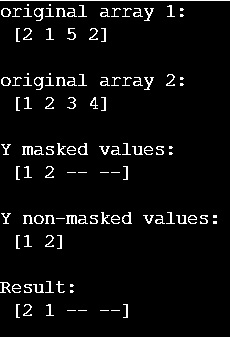# How to apply a mask from one array to another array in Python?

Learn, how to apply a mask from one array to another array in Python NumPy? By Pranit Sharma Last updated : April 04, 2023

Suppose that we are given two numpy arrays x and y, we need to apply a mask on y and then apply the mask on x based on y to create a new x.

## Mask an array using another array

To mask an array using another array, we use numpy.ma.masked_where() to apply the mask on y and then we will use ma.compressed() on the result to return the non-masked data. Once the masking is done on y, we will mask on x by giving a condition for y.

Let us understand with the help of an example,

## Python code to mask an array using another array

```# Import numpy
import numpy as np

# Creating numpy arrays
x = np.array([2,1,5,2])
y = np.array([1,2,3,4])

# Display original arrays
print("original array 1:\n",x,"\n")
print("original array 2:\n",y,"\n")

n_y = np.ma.compressed(m_y)

# masking x based on y

# Display result
print("Result:\n",res)
```

### Output